# Working with Linear Equations Lesson Plans Chapter Exam

Exam Instructions:

Choose your answers to the questions and click 'Next' to see the next set of questions. You can skip questions if you would like and come back to them later with the yellow "Go To First Skipped Question" button. When you have completed the practice exam, a green submit button will appear. Click it to see your results. Good luck!

### Page 1

#### Question 3 3. If the graph below has the equation Ax + By = -4, what must be true about A and B?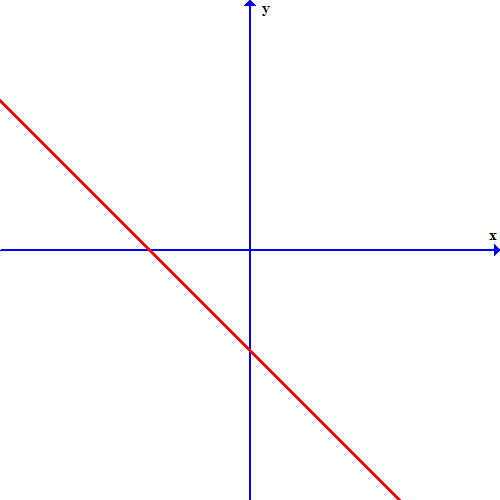### Page 3

#### Question 13 13. If the graph below was written in the slope-intercept form, which of the following would be true?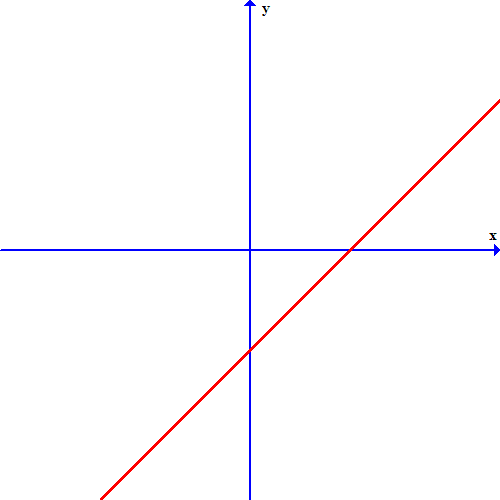#### Question 15 15. Which linear equation is shown below?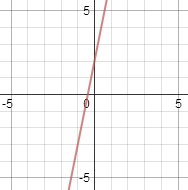### Page 4

#### Question 18 18. Which of these equations represents a line that is perpendicular to the line in the graph below?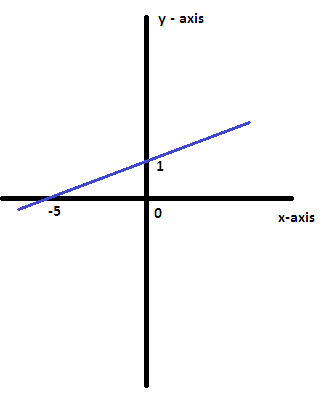#### Question 20 20. What is the slope-intercept form equation of the graph below?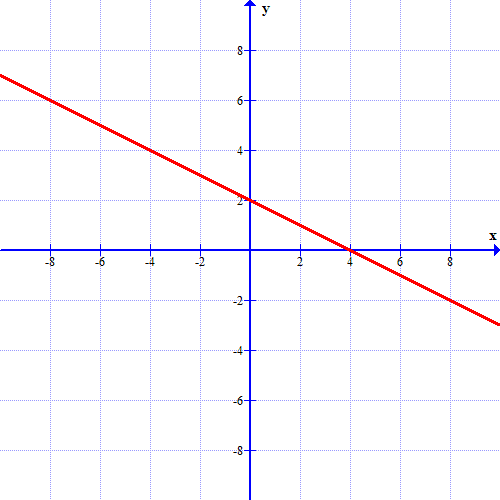### Page 5

#### Question 25 25. Which one of the following equations could work for the following graph?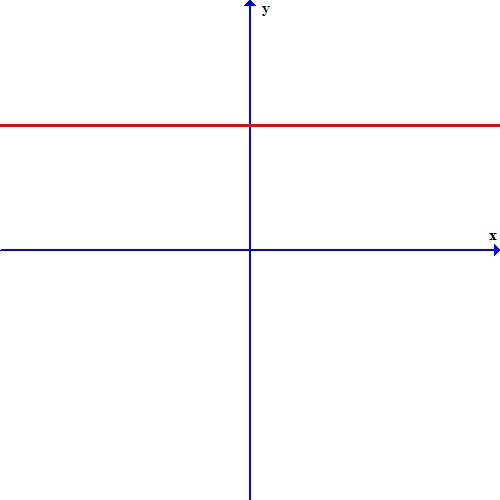### Page 6

#### Working with Linear Equations Lesson Plans Chapter Exam Instructions

Choose your answers to the questions and click 'Next' to see the next set of questions. You can skip questions if you would like and come back to them later with the yellow "Go To First Skipped Question" button. When you have completed the practice exam, a green submit button will appear. Click it to see your results. Good luck!

Support